Students can download 5th Maths Term 1 Chapter 1 Geometry Ex 1.3 Questions and Answers, Notes, Samacheer Kalvi 5th Maths Guide Pdf helps you to revise the complete Tamilnadu State Board New Syllabus, helps students complete homework assignments and to score high marks in board exams.

## Tamilnadu Samacheer Kalvi 5th Maths Solutions Term 1 Chapter 1 Geometry Ex 1.3

Question 1.
The angles below 90° and above 0° are called as _________
acute angleQuestion 2.
The angles below 180° and above 90° are called as __________
obtuse angle.

Question 3.
By joining two right angles ________ angle is formed
straight angle

Question 4.
The obtuse angle in ΔABC is ________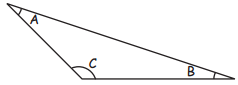a. ∠A
b. ∠B
c. ∠C
c) ∠C

Question 5.
Hand of a clock at 3.20 shows ________ angle.
acuteQuestion 6.
In the following letters, which one forms the right angle in it?
a. L
b. K
c. Z
d. N
a. L

Question 7.
Circle the right angle.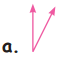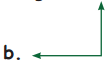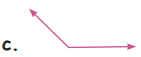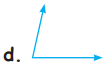Question 8.
The angle shown in this picture is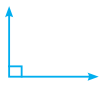a. more than 120°
b. Less than 45°
c. more than 180°
d. 90°